JavaTest1
What is your LAST Name? *
What is your FIRST Name? *
FIGURE 1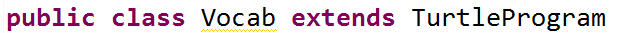The code in figure 1 above is *
1 point
FIGURE 2The code in figure 2 above is *
1 point
FIGURE 3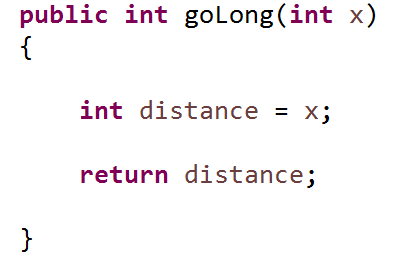The code in figure 3 above is *
1 point
Using figure 3, the return type is *
1 point
Using figure 3 above, what variable type does the parameters require? *
1 point
FIGURE 4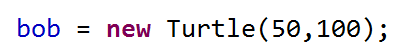Choose ALL that identify the code in figure 4 *
0 points
Required
FIGURE 5The code in figure 5... *
1 point
If we were to place the code in figure 5 in a method, what would be the BEST way to do it? *
1 point
FIGURE 6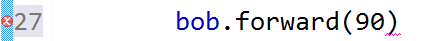The code in figure 6 has an error because... *
1 point
The code in figure 6 is an example of *
1 point
Primitive variable types are those that *
1 point
Integers are *
1 point
FIGURE 7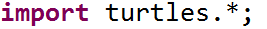Which BEST describes the purpose of the code in figure 7? *
1 point
FIGURE 8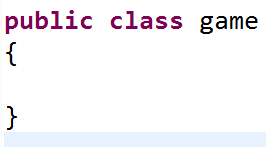The code in figure 8... *
1 point
I feel like I am learning in this class and that I am capable of being succesful in here *